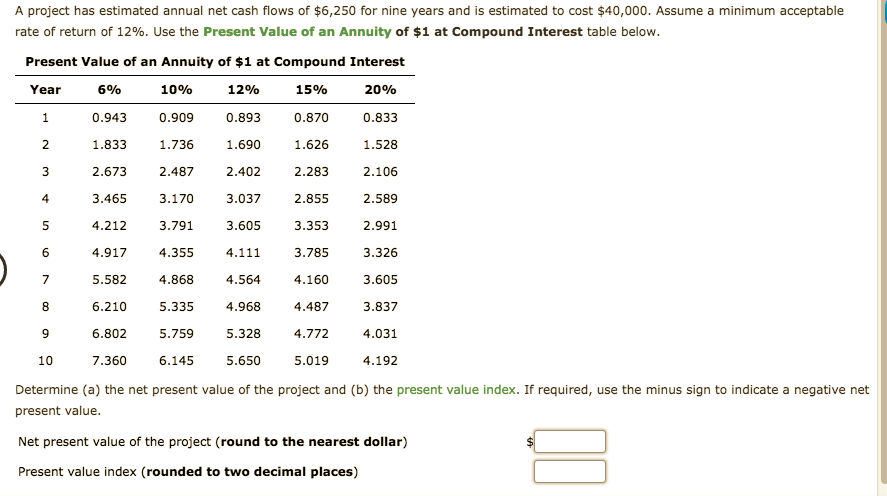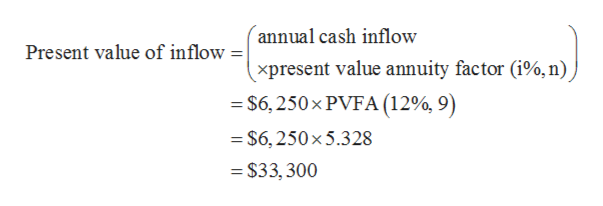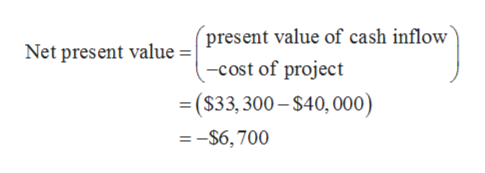# A project has estimated annual net cash flows of \$6,250 for nine years and is estimated to cost \$40,000. Assume a minimum acceptablerate of return of 12%. Use the Present Value of an Annuity of \$1 at Compound Interest table below.Present Value of an Annuity of \$1 at Compound InterestYear10%12%15%0.9430.9090.8930.8700.8331.8331.7361.6901.6261.5282.10632.6732.4872.4022.28343.4653.1703.0372.8552.5893.3534.2123.7913.6052.9913.3266.4.9174.3554.1113.7853.6055.5824.8684.5644.1606.2105.3354.9684.4873.8376.8025.7595.3284.7724.0315.650107.3606.1455.0194.192Determine (a) the net present value of the project and (b) the present value index. If required, use the minus sign to indicate a negative netpresent value.Net present value of the project (round to the nearest dollar)Present value index (rounded to two decimal places)

Question
1 viewshelp_outlineImage TranscriptioncloseA project has estimated annual net cash flows of \$6,250 for nine years and is estimated to cost \$40,000. Assume a minimum acceptable rate of return of 12%. Use the Present Value of an Annuity of \$1 at Compound Interest table below. Present Value of an Annuity of \$1 at Compound Interest Year 10% 12% 15% 0.943 0.909 0.893 0.870 0.833 1.833 1.736 1.690 1.626 1.528 2.106 3 2.673 2.487 2.402 2.283 4 3.465 3.170 3.037 2.855 2.589 3.353 4.212 3.791 3.605 2.991 3.326 6. 4.917 4.355 4.111 3.785 3.605 5.582 4.868 4.564 4.160 6.210 5.335 4.968 4.487 3.837 6.802 5.759 5.328 4.772 4.031 5.650 10 7.360 6.145 5.019 4.192 Determine (a) the net present value of the project and (b) the present value index. If required, use the minus sign to indicate a negative net present value. Net present value of the project (round to the nearest dollar) Present value index (rounded to two decimal places) fullscreen
check_circle

star
star
star
star
star
1 Rating
Step 1

Net present value of a project is calculated by deducting the cost of project from the present value of cash inflows. A project is selected if it has positive net present value and vice-a-versa.

Step 2

Cost of project: \$40,000

Annual cash flow: \$6,250

Time: 9 years

Interest rate: 12%

a)

Present value of cash inflow is calculated as follows:help_outlineImage Transcriptioncloseannual cash inflow Present value of inflow = xpresent value annuity factor (i%, n) = \$6, 250× PVFA (12%, 9) = \$6, 250×5.328 = \$33, 300 fullscreen
Step 3

Net present value of the project ...help_outlineImage Transcriptionclosepresent value of cash inflow -cost of project Net present value = = (\$33, 300 – \$40, 000) =-\$6,700 fullscreen

### Want to see the full answer?

See Solution

#### Want to see this answer and more?

Solutions are written by subject experts who are available 24/7. Questions are typically answered within 1 hour.*

See Solution
*Response times may vary by subject and question.
Tagged in